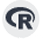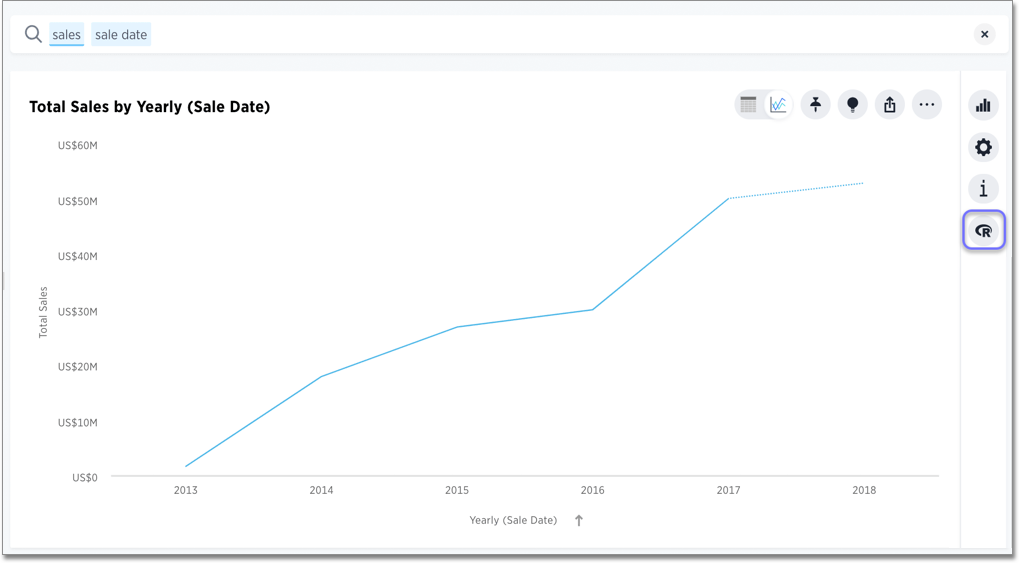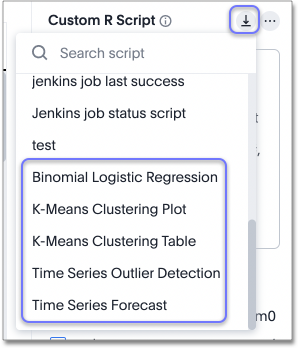# Run prebuilt R scripts on answers

Anyone with R privileges can run an R analysis in ThoughtSpot using provided scripts. You don’t need to be an expert.

If you have R privileges on your ThoughtSpot instance, you can run R analyses on search results, and save and share the resulting visualization with others. The users you share visualizations with do not need R privileges.

 We support R only in Falcon deployments (data imported into ThoughtSpot), and R integration is disabled by default. It is not available when creating connections and linking to external databases in real time.

## Run an R analysis using a pre-built script

1. Select the R iconon the toolbar for any search result (answer).From here, you can write a custom script, or load a pre-built or ThoughtSpot-provided script.

2. Select the Load script template icon in the R analysis panel, next to the Custom R Script panel.This brings up a list of pre-built scripts, including the scripts provided by ThoughtSpot and any scripts created by programmers on your team.

3. Select a script.

4. Choose the columns you want to include in the analysis.

For example, if you select one of the ThoughtSpot-provided time series scripts, the comment at the top of the script provides guidance on what columns to select:

``` # NOTE: Only two columns are needed for this analysis.
# The first column should represent some date column
# and the second should be some numeric measure column.```
5. Choose the output file type (PNG or CSV).

Note that the output file type must match the script. To ensure this, scroll through the script to identify whether it is coded to produce graphical (PNG) or tabular (CSV) output. The time series scripts are both set up to produce graphical output, as indicated by a line like this at the end of the scripts.

``` png(#output_file#, width=1000);
print(img);```
6. Select Run Analysis to execute the script.

## Diverging Bars Example

Here is an example of taking a script found online and repurposing it for a use case in ThoughtSpot.

Consider this script, found on this website of Top 50 ggplot2 Visualizations - The Master List (With Full R Code). A direct link to this script is here.

```library(ggplot2)
theme_set(theme_bw())

# Data Prep
mtcars\$`car name` <- rownames(mtcars)  # create new column for car names
mtcars\$mpg_z <- round((mtcars\$mpg - mean(mtcars\$mpg))/sd(mtcars\$mpg), 2)  # compute normalized mpg
mtcars\$mpg_type <- ifelse(mtcars\$mpg_z < 0, "below", "above")  # above / below avg flag
mtcars <- mtcars[order(mtcars\$mpg_z), ]  # sort
mtcars\$`car name` <- factor(mtcars\$`car name`, levels = mtcars\$`car name`)  # convert to factor to retain sorted order in plot.

# Diverging Barcharts
ggplot(mtcars, aes(x=`car name`, y=mpg_z, label=mpg_z)) +
geom_bar(stat='identity', aes(fill=mpg_type), width=.5)  +
scale_fill_manual(name="Mileage",
labels = c("Above Average", "Below Average"),
values = c("above"="#00ba38", "below"="#f8766d")) +
labs(subtitle="Normalised mileage from 'mtcars'",
title= "Diverging Bars") +
coord_flip()```

In this script, `mtcars` is replaced with references to our phone sales (`df\$Sales`) and `car name` is replaced with `Device Name`. The script uses the ThoughtSpot data frame object (`df`), and adds two lines at the end to specify output type as a `png` image.

```library(ggplot2)
theme_set(theme_bw())

# Data Prep
df\$sales_z <- round((df\$Sales - mean(df\$Sales))/sd(df\$Sales), 2)  # compute normalized mpg
df\$sales_type <- ifelse(df\$sales_z < 0, "below", "above")  # above / below avg flag
df <- df[order(df\$sales_z), ]  # sort
df\$`Device Name` <- factor(df\$`Device Name`, levels = df\$`Device Name`)  # convert to factor to retain sorted order in plot.

# Diverging Barcharts
img <- ggplot(df, aes(x=`Device Name`, y=sales_z, label=sales_z)) +
geom_bar(stat='identity', aes(fill=sales_type), width=.5)  +
scale_fill_manual(name="Sales",
labels = c("Above Average", "Below Average"),
values = c("above"="#00ba38", "below"="#f8766d")) +
labs(subtitle="Normalised Sales for Phones",
title= "Diverging Bars") +
coord_flip()
png(#output_file#, width=1000, height=1000)
print(img)```

Related information